Get instant live expert help with Excel or Google Sheets“My Excelchat expert helped me in less than 20 minutes, saving me what would have been 5 hours of work!”

#### Post your problem and you’ll get expert help in seconds.

Your message must be at least 40 characters
Our professional experts are available now. Your privacy is guaranteed.
All articles PIVOT TABLE How to Group Pivot Table Data in Excel

# How to Group Pivot Table Data in Excel

We can use a PivotTable to GROUP A SET OF DATA like dates, months, years, quarters, etc. This enables us to analyze, summarize, calculate, and visualize trends, comparisons, and patterns in our data. The steps below will walk through the process of Grouping Pivot Table Data.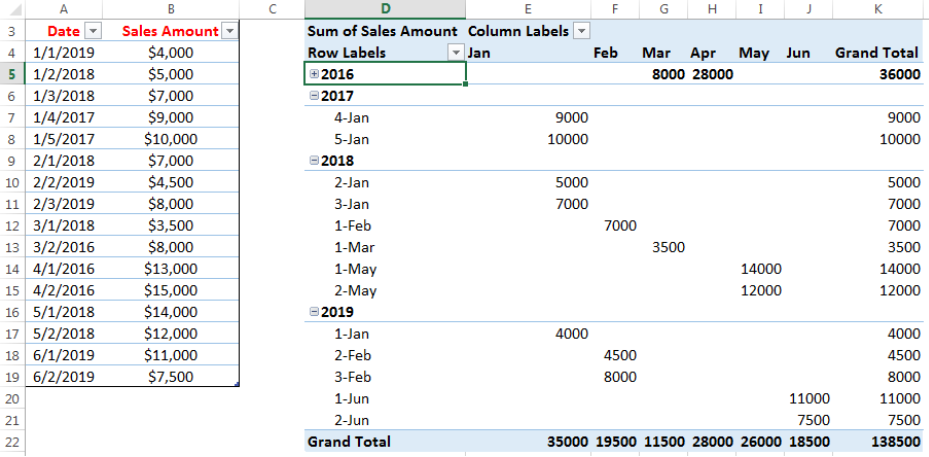Figure 1- How to Group Pivot Table Data

## Setting up the Data

• We will open a New excel sheet
• We will input dates when sales were made in Column A and the corresponding Sales Amount in Column B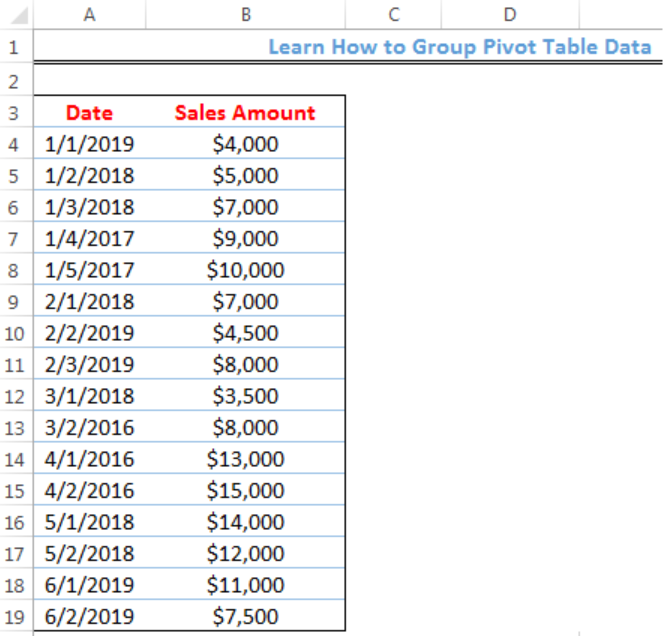Figure 2- Setting up the Data

• We will click on anywhere on the table, click on the Insert tab, and click on Table as shown in figure 3Figure 3- Putting the data in a Table

• We will click on OK on the dialog box that appears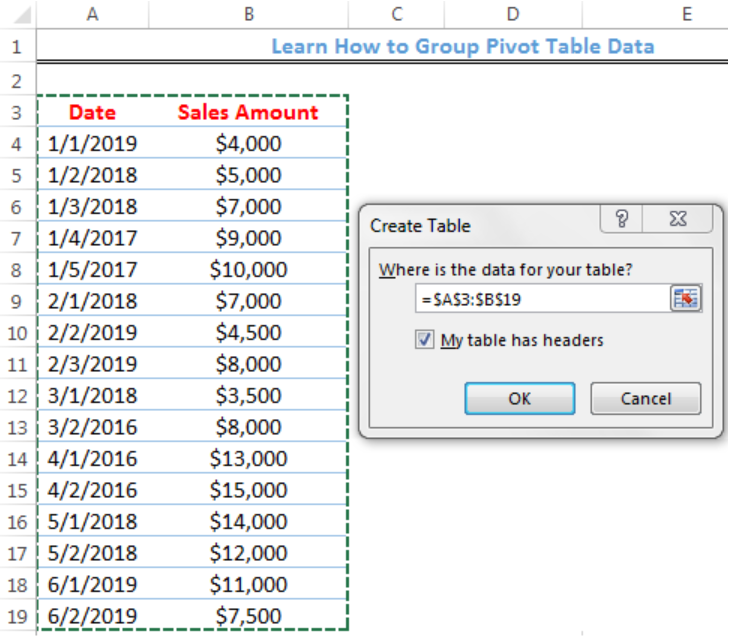Figure 4- Create Table Dialog Box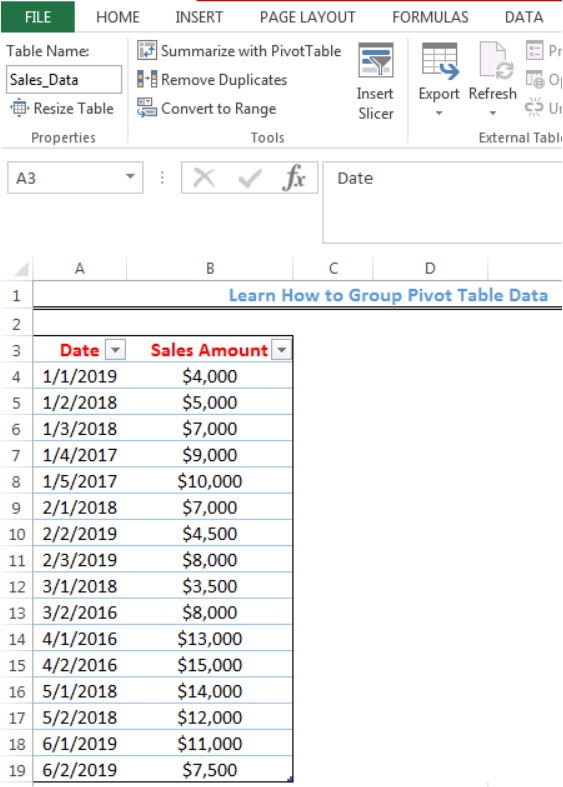Figure 5- Created Table

• We will click on the box below Table Name under File in Figure 5 and name the Table as Sales_Data
• We will press enter after inserting the name
• Now, we will create a Pivot Table with the Data

## Creating the Pivot Table

• We will click on anywhere on the table
• We will click on the Insert tab and click on Pivot Table as shown in figure 3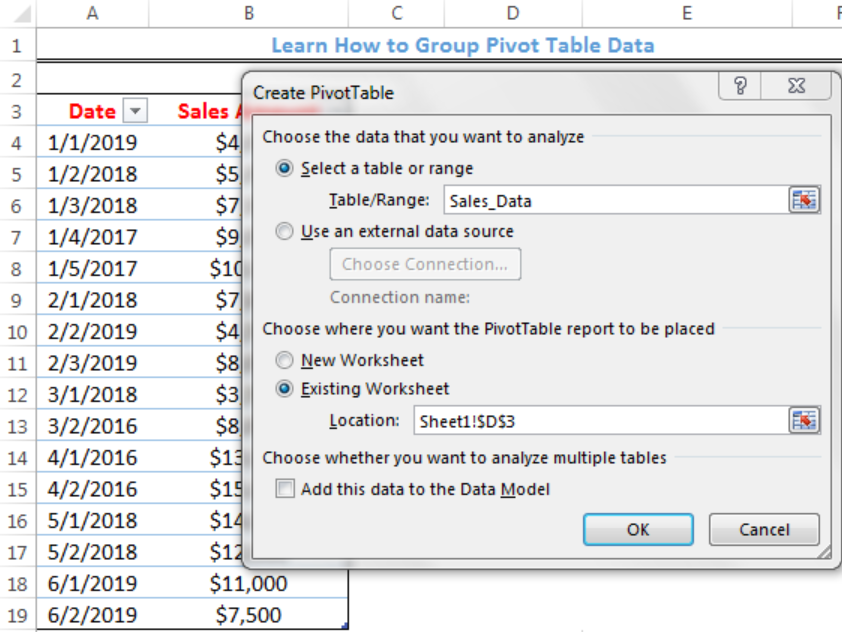Figure 6- Creating the Pivot Table

• We will click on existing worksheet and specify the Location where the Pivot table will start from (Sheet1!\$D\$3)
• We will click on OK
• We will select the fields we want to add to the Pivot Table (Dates and Sales Amount)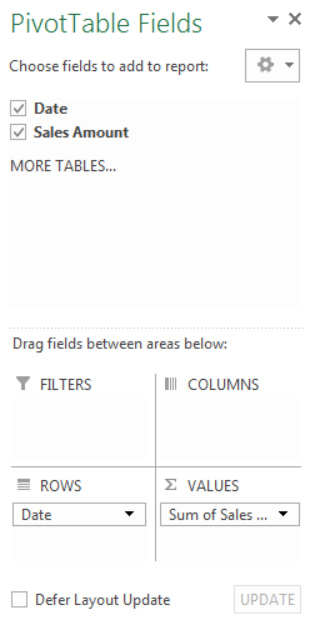Figure 7- Selecting Pivot Table Fields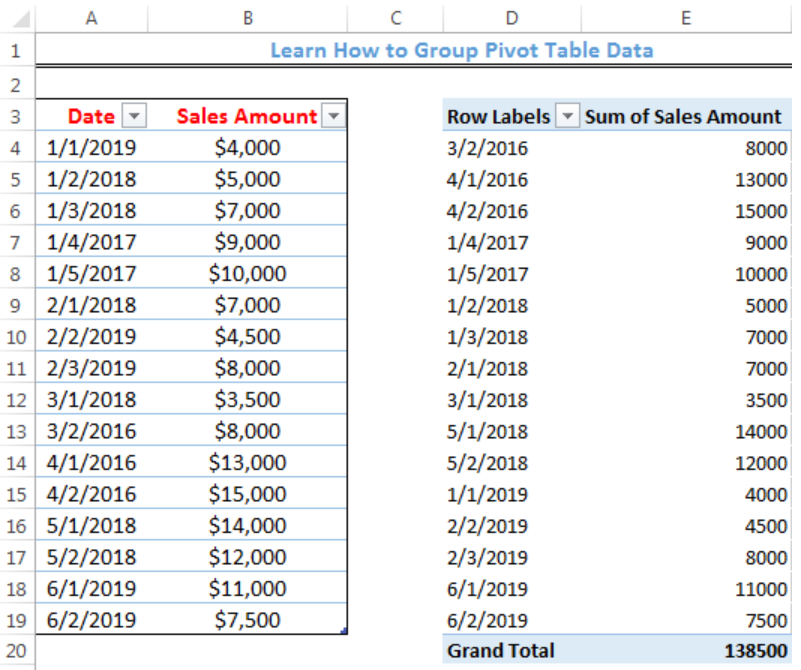Figure 8- Pivot Table

## Grouping the Pivot Table Data by Year

• We will click on any date within the Pivot Table
• We will right-click and click on GROUP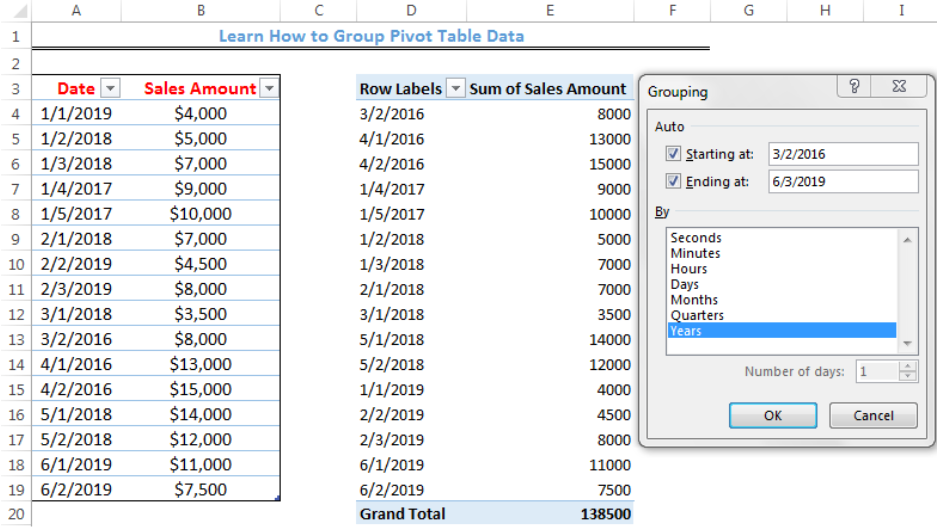Figure 9- Grouping Dialog box

• We will click OK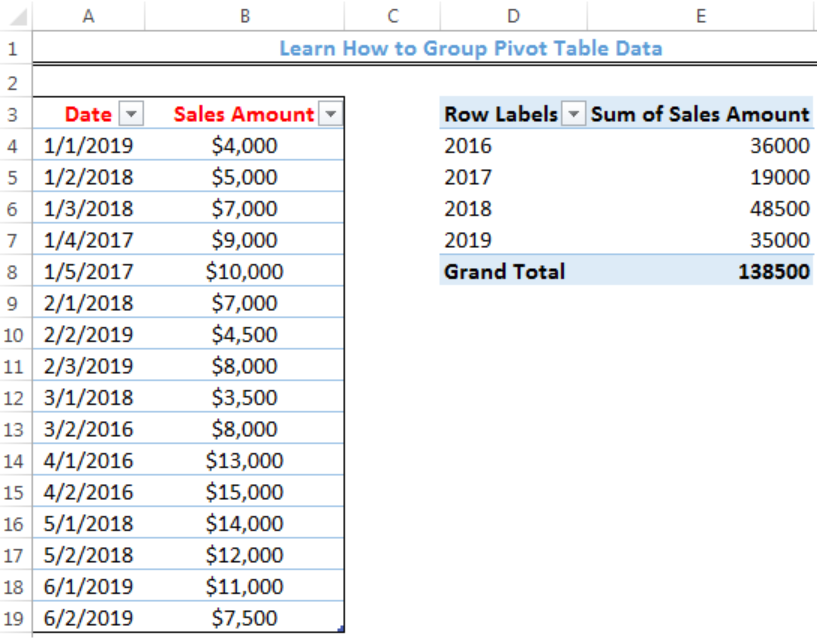Figure 10- Pivot Table Grouped by Year

## Grouping the Pivot Table Data by Days

In the same way, AS DESCRIBED FOR GROUPING BY YEAR, we can select days as shown in figure 10, specify the number of days, and click OK.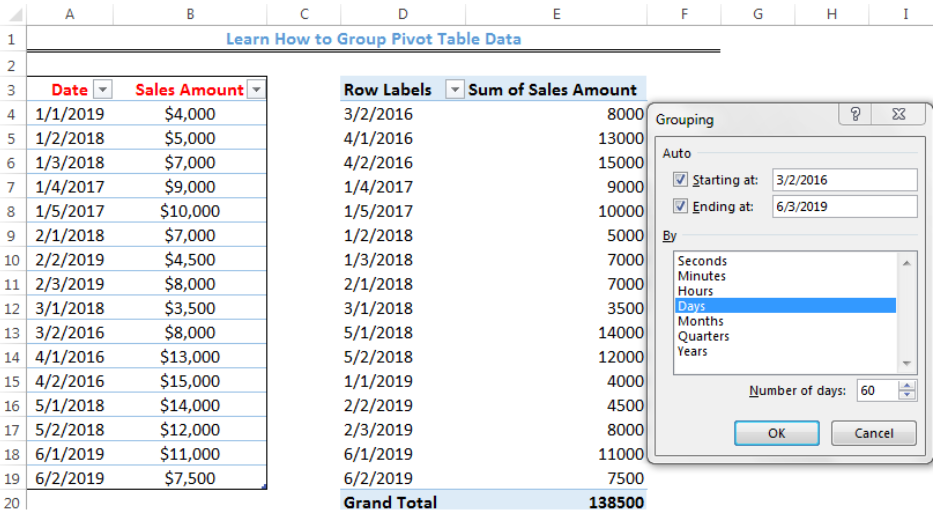Figure 11- Grouping Dialog box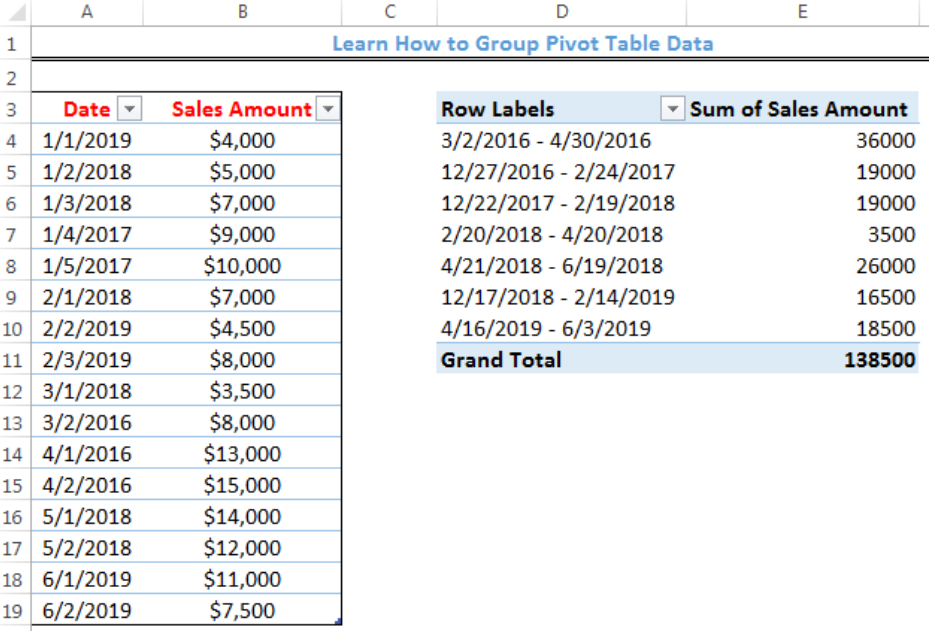Figure 12- Pivot Table Grouped by Days

• The same procedure can be followed to group pivot table data by months and quarters.

## Grouping the Pivot Table Data by Days, Months, and Year

• We can group our Pivot Table by clicking on the three options; Days, Months, and Year on the grouping dialog box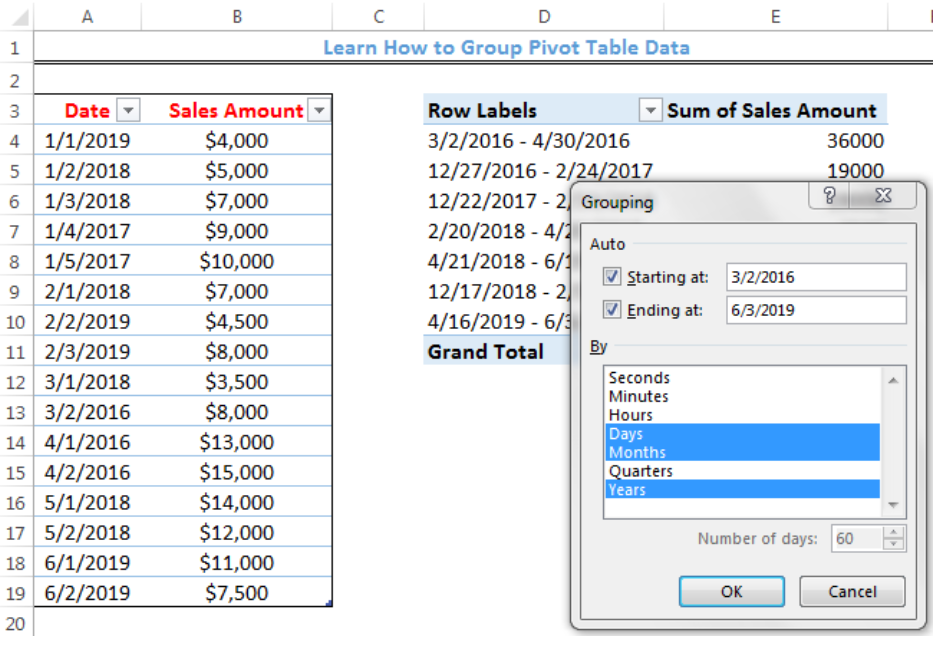Figure 13- Grouping the Pivot Table Data by Days, Months, and Year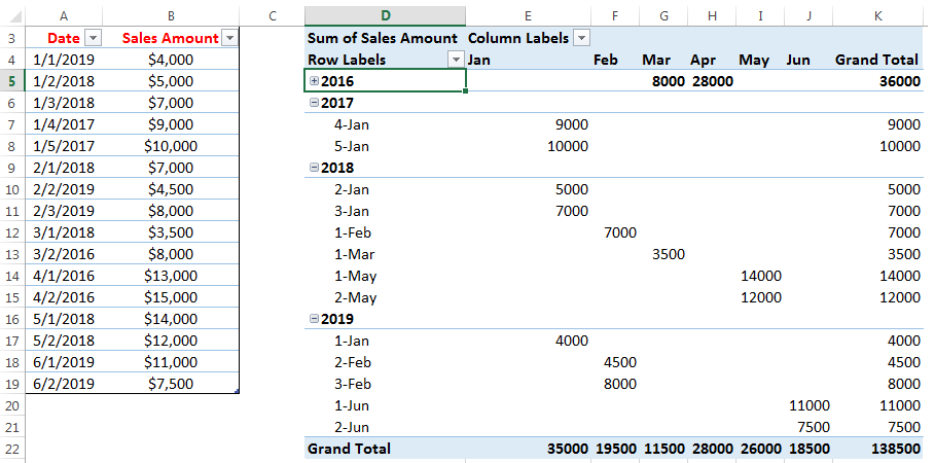Figure 14- Grouped Pivot Table Data by Days, Months, and Year

• We should note that we have clicked on the dash () sign for 2016 to hide the details for us to have a full view of the Pivot table. Also, the arrangement of the Columns and rows is by dragging the Pivot Table Fields to the locations as shown in Figure 15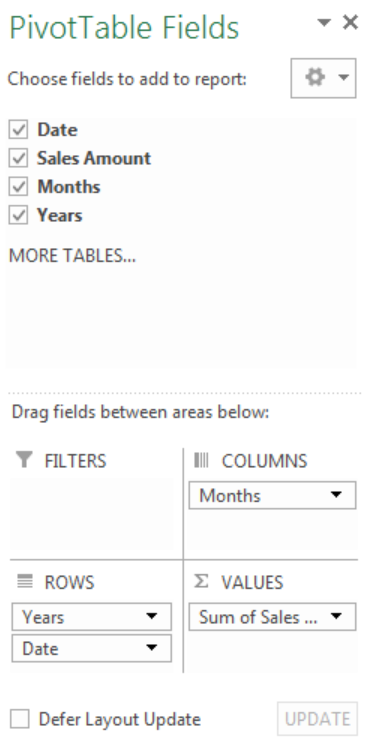Figure 15: Pivot Table fields for Grouping by Days, Months, and Year

## Note

• Double-clicking on any of the Row labels will drop-down or hide the details for that year

## Instant Connection to an Expert through our Excelchat Service

Most of the time, the problem you will need to solve will be more complex than a simple application of a formula or function. If you want to save hours of research and frustration, try our live Excelchat service! Our Excel Experts are available 24/7 to answer any Excel question you may have. We guarantee a connection within 30 seconds and a customized solution within 20 minutes.

Solution examplesI need a formula that looks up if the date (which looks like "8/1/2018 8:05:36 AM") in Column "C" contains year 2018. And then populates "2018" in Column D, if the date does not contain "2018" then enter False in Column D.
Solved by F. H. in 20 minscan you teach me the steps to create a nested if function, and to nest an AND function inside of an IF function?
Solved by A. Q. in 22 minsa date formula that results in showing the date as 29/12/00 (date format as dd/mm/yy) - use column BA to calculate 364 days (same date format) in column BS, if BA blank, then calculate 364 days using column AX. Results for column BA are correct. However, if column BA is empty, then it should calculate using column AX, which has data, but the result is always 29/12/00, regardless of the date in column AX. I have used this formula with success in another workbook, but this file wont work! Formula: =IF(AND(LEN(\$BA74)=0,LEN(\$AX74)=0),"",IF(LEN(\$BA74)=0,DATE(YEAR(\$AX74),MONTH(\$AX74),DAY(\$AX74)+364),DATE(YEAR(\$BA74),MONTH(\$BA74),DAY(\$BA74)+364)))
Solved by A. L. in 60 minsneed help in this =IF(C2="","",IF(ISBLANK(IF(C2="yes",HLOOKUP(VALUE(MONTH(A2) & "/" & DAY(A2) & "/" & YEAR(A2)),Sheet2!\$B\$1:\$AA\$1000,2,FALSE),IF(C2="no",HLOOKUP(VALUE(MONTH(A2) & "/" & DAY(A2) & "/" & YEAR(A2)),Sheet2!\$B\$1:\$AA\$1000,3,FALSE),""))),"NOT YET",IF(C2="yes",HLOOKUP(VALUE(MONTH(A2) & "/" & DAY(A2) & "/" & YEAR(A2)),Sheet2!\$B\$1:\$AA\$1000,2,FALSE),IF(C2="no",HLOOKUP(VALUE(MONTH(A2) & "/" & DAY(A2) & "/" & YEAR(A2)),Sheet2!\$B\$1:\$AA\$1000,3,FALSE),""))))
Solved by V. Q. in 11 minsneed help =IF(C2="","",IF(ISBLANK(IF(C2="yes",HLOOKUP(VALUE(MONTH(A2) & "/" & DAY(A2) & "/" & YEAR(A2)),Sheet2!\$B\$1:\$AA\$1000,2,FALSE),IF(C2="no",HLOOKUP(VALUE(MONTH(A2) & "/" & DAY(A2) & "/" & YEAR(A2)),Sheet2!\$B\$1:\$AA\$1000,3,FALSE),""))),"NOT YET",IF(C2="yes",HLOOKUP(VALUE(MONTH(A2) & "/" & DAY(A2) & "/" & YEAR(A2)),Sheet2!\$B\$1:\$AA\$1000,2,FALSE),IF(C2="no",HLOOKUP(VALUE(MONTH(A2) & "/" & DAY(A2) & "/" & YEAR(A2)),Sheet2!\$B\$1:\$AA\$1000,3,FALSE),""))))
Solved by G. S. in 9 mins## Subscribe to Excelchat.co